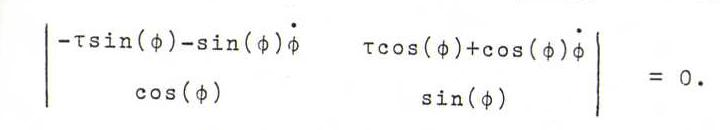### DIFFERENTIAL GEOMETRY COURSE

8. EVOLVENT AND EVOLUTE

Definition 76: Let C be a curve. Let K be a curve such that the tangents to C are normals of K. Then we call K an evolvent of C, and C an evolute of K.

Proposition 77: Every curve has ∞1 evolvents and ∞1 evolutes.

Proof : Use a parametrisation of C according to arc length: x(s). Then an evolvent K has parametrisation y(s)=x(s)+λ(s)t(s) (s is not arc length of K).
Now t(s) must be perpendicular to y '(s), where y '(s) = x .(s) + λ .(s)t(s) + λ(s)κ(s)n(s) = (1 + λ .(s))t(s) + λ(s)κ(s)n(s).
So 1 + λ .(s) = 0 and s + λ(s) = c. Then we find for each constant c an evolvent Kc with parametrisation y(s) = x(s) + (c-s)t(s).

On the other hand, use a parametrisation K according to arc length: x(s). A normal vector has the form n(s)cos(φ(s)) + b(s)sin(φ(s)).
Then a corresponding evolute C has the following parametrisation (where s is not arc length): y(s)=x(s)+λ(s)(n(s)cos(φ(s)) + b(s)sin(φ(s))).
Now y '(s) must be a scalar multiple of n(s)cos(φ(s)) + b(s)sin(φ(s)), where
y '(s) = t + λ .(n cos(φ) + b sin(φ)) + λ(n .cos(φ) + b .sin(φ) - n sin(φ)φ . + b cos(φ)φ .) =
t(1-λκ cos(φ)) + n .cos(φ) - λτ sin(φ) - λ sin(φ)φ .) + b .sin(φ) + λτ cos(φ) + λ cos(φ)φ .).
So the following must hold: first, λ = (κ cos(φ))-1, and, second,Hence φ . = - τ, so φ = φo - ∫0s τ(u) du.
With each choice of φo we find an evolute C.

Problem 78:
Show that we get the normals of a curve K corresponding to an evolute C from the normals of another evolute C' by rotating each of them in its normal plane over a fixed angle.
Furthermore, show that the contact point y(s) of the evolute C lies on the curvature axis of the point x(s) of K.
Finally show that the principal normals of a curve K are the tangents to a curve C (so that C is the "envelope" of these tangents) if and only if τ=0, so if K is planar. Then C is the locus of the centers of curvature of K.

Problem 79: Show that the tangents to a circular helix intersect each plane perpendicular to the axis in the points of an evolvent of the circle that is the intersection of this plane and the cilinder on which the helix is lying.

Problem 80: Given a cycloid (see 18, 28), determine its evolute, and show this is also a cycloid.